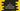In this tutorial, we will learn how to add two numbers using addresses in C programming language. Mainly we will learn how to access the values of one address in C. Let’s take a look into the algorithm first :

### Algorithm :

1. First ask the user to input the numbers and store them in two integer variables.
2. Get the address of both numbers and store them in two different variables.
4. Add both of them and store it in a different variable.
5. Print out the result.

### C program :

``````#include <stdio.h>

int main()
{
//1
int first, second;

//2

//3
int sum;

//4
printf("Enter the first and second number : \n");

//5
scanf("%d %d", &first, &second);

//6

//7

//8
printf("The sum of %d and %d is %d \n", first, second, sum);
return 0;
}``````

### Explanation :

_The commented numbers in the above program denotes the step number below : _

1. Create two variables to store the first and second number : first and second.
``````Enter the first and second number :